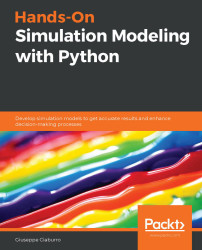•#### Hands-On Simulation Modeling with Python#### Overview of this book

Simulation modeling helps you to create digital prototypes of physical models to analyze how they work and predict their performance in the real world. With this comprehensive guide, you'll understand various computational statistical simulations using Python. Starting with the fundamentals of simulation modeling, you'll understand concepts such as randomness and explore data generating processes, resampling methods, and bootstrapping techniques. You'll then cover key algorithms such as Monte Carlo simulations and Markov decision processes, which are used to develop numerical simulation models, and discover how they can be used to solve real-world problems. As you advance, you'll develop simulation models to help you get accurate results and enhance decision-making processes. Using optimization techniques, you'll learn to modify the performance of a model to improve results and make optimal use of resources. The book will guide you in creating a digital prototype using practical use cases for financial engineering, prototyping project management to improve planning, and simulating physical phenomena using neural networks. By the end of this book, you'll have learned how to construct and deploy simulation models of your own to overcome real-world challenges.
PrefaceSection 1: Getting Started with Numerical SimulationFree Chapter
Chapter 1: Introducing Simulation ModelsChapter 2: Understanding Randomness and Random NumbersChapter 3: Probability and Data Generation ProcessesSection 2: Simulation Modeling Algorithms and TechniquesChapter 4: Exploring Monte Carlo SimulationsChapter 5: Simulation-Based Markov Decision ProcessesChapter 6: Resampling MethodsChapter 7: Using Simulation to Improve and Optimize SystemsSection 3: Real-World ApplicationsChapter 8: Using Simulation Models for Financial EngineeringChapter 9: Simulating Physical Phenomena Using Neural NetworksChapter 10: Modeling and Simulation for Project ManagementChapter 11: What's Next?Other Books You May Enjoy# Explaining probability concepts

If we take a moment to reflect, we'll notice that our everyday lives are full of probabilistic considerations, although not necessarily formalized as such. Examples of probabilistic assessments include choosing to participate in a competition given the limited chance of winning, the team's predictions of winning the championship, statistics that inform us about the probability of death from smoking or failure to use seat belts in the event of a road accident, and the chances of winning in games and lotteries.

In all situations of uncertainty, there is basically a tendency to give a measure of uncertainty that, although indicated in various terms, expresses the intuitive meaning of probability. The fact that probability has an intuitive meaning also means that establishing its rules can, within certain limits, be guided by intuition. However, relying completely on intuition can lead to incorrect conclusions. To avoid reaching incorrect...